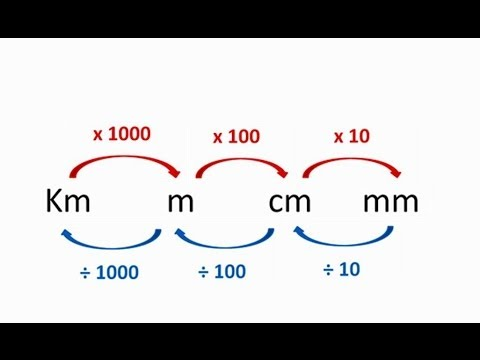# Maths

It's time to apply some of our knowledge and jog our memory today Year 4, as we look at converting units of measurement for length. What are units of measurement for length, though? Have a watch of this BBC bitesize clip:

https://www.bbc.co.uk/bitesize/topics/z4nsgk7/articles/zqf4cwx

So how do we convert from one to the other? The answer is in multiplying and dividing by 10, 100 and 1000. We've worked hard on our place value so we should have a fairly good idea of how to do that.So, if we have 5 km and wanted that in m, we have to x 1000 to get 5000m

If we had 8000 m and wanted that in km, we'd go the other way - divide by 1000 to get 8 km. Remember - none of the digits change, they just move place value.

Same with metres into centimetres.

If we had 14m, multiply by 100 and we get 1400 cm.

If we had 5200 cm, divide by 100 and we get 52m.

See how it works? Log onto Mathletics where you'll have - Converting cm and mm (and a few more, if you're up to the challenge). Good luck!

Top